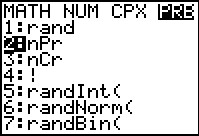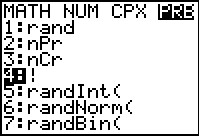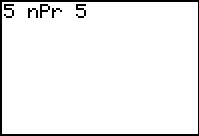••• ##### Device
• TI-83 Plus Family
• TI-84 Plus
• TI-84 Plus Silver Edition
•TI-84 Plus C Silver Edition
•TI-84 Plus CE
• ##### Report an Issue

Algebra II: Permutations
by Texas Instruments#### Overview

Students are led through the development of the formula for finding n objects taken n at a time and n objects taken r at a time.

#### Key Steps

•Students use their calculator to investigate factorials and the fundamental counting principle. They evaluate given expressions by using the factorial symbol "!".

•In the next problem, students find the number of permutations of n objects taken n at a time.

•Students go over the definition of a permutation and use the calculator to solve given expressions. They use the nPr command to find the number of permutations.